• 本文详细介绍Pandas数据结构DataFrame和Series的常用方法。欢迎微信随缘关注@pythonic生物人本文可以学到什么？1、pandas简介 2、pandas数据结构之-Series pandas.Series快速创建 pandas.Series取出所有值：values ...本文详细介绍Pandas数据结构DataFrame和Series的常用方法。

欢迎微信随缘关注@pythonic生物人
本文可以学到什么？

1、pandas简介

2、pandas数据结构之-Series
pandas.Series快速创建
pandas.Series取出所有值：values
pandas.Series取出索引：index
pandas.Series类似于numpy.ndarry的性能
pandas.Series通过索引值取值
pandas.Series类似字典（dict）的性能

3、pandas数据结构之-DataFrame
DataFame创建
pandas.DataFrame中取列操作
pandas.DataFrame中取某一列
pandas.DataFrame中取某几列-方法1
pandas.DataFrame中取某几列-方法2

pandas.DataFrame中取行操作
pandas.DataFrame中取某一行
pandas.DataFrame中取某几行
pandas.DataFrame中按一个条件过滤行
pandas.DataFrame中按多个条件过滤行
pandas.DataFrame中结合map 和lambda函数过滤行
pandas.DataFrame中结合isin过滤行
pandas.DataFrame中取某几行中的某几列

pandas.DataFrame中替换值：where方法
pandas.DataFrame中查找某个位置的值：lookup方法
pandas.DataFrame中末尾新增加列
pandas.DataFrame中指定位置新增加列：insert方法
pandas.DataFrame中删除列：del方法
pandas.DataFrame复制：copy方法
Series、pandas.DataFrame中某列唯一值的个数：unique
pandas.DataFrame统计列中每个元素出现的频次：value_counts方法
pandas.DataFrame按照某几列分组并统计：groupby+count
pandas.DataFrame按照某列分组并求和
pandas.DataFrame按照某列分组并取出某个小组：groupby+get_group

pandas.DataFrame排序
pandas.DataFrame按照行标签或者列标签排序：sort_index方法
pandas.DataFrame按照某列值排序：sort_values方法by参数
pandas.DataFrame描述统计

pandas.DataFrame获取行列标签/表头名称


1、pandas简介
pandas模块是在numpy的基础上构建的，速度快（层算法都用 Cython优化过）、有大量直接操作每个元素的函数；常常和Matplotlib，seaborn，statsmodels，scikit-learn等包一起使用；相比于numpy，pandas更擅长处理表格型（Tabular data）数据，表格中每列数据类型可以不一致，numpy数组规定数据类型需要一致；主要有两种数据结构：一维Series（类似numpy.ndarray）带行标签，二维DataFrame（比R中data.frame更丰富），同时带行标签和列标签；关于pandas的使用可以在这里搜索，点击戳：https://pandas.pydata.org/pandas-docs/stable/reference/index.html 。python社区默认将pandas模块简写为pd，导入模块的时候要一起导入pandas的两种数据结构：Series和DataFrame：

In : import pandas as pd
In : from pandas import Series,DataFrame#导入两种数据结构。


2、pandas数据结构1-Series
Series是一个自带标签的一维数组（ one-dimensional labeled array），结构图如下：Series具有大量属性和函数：

In : len(dir(Series))
Out: 471#方法多大400多种，详细使用如下：


详细使用请戳我哦
pandas.Series快速创建
语法：

pd.Series(data=None, index=None, dtype=None, name=None, copy=False, fastpath=False)
data：python中的字典、可迭代对象、numpy.ndarry或者标量值
index：可选项，默认为列表[0, ..., len(data) - 1]，可以自己传入
dtype：可选项，设置数据类型

In : data = pd.Series([1,2,3,4,5])#不指定索引值，默认索引值为[0, ..., len(data) - 1]
In : data
Out:
0 1
1 2
2 3
3 4
4 5
dtype: int64

#pandas.Series自定义索引值
In : data1 = pd.Series([1,2,3,4,5],index=list('abcde'))
In : data1
Out:
a 1
b 2
c 3
d 4
e 5
dtype: int64


pandas.Series取出所有值：values
返回一个数组。

In : data1.values
Out: array([1, 2, 3, 4, 5], dtype=int64)


pandas.Series取出索引：index
返回一个数组

In : data1.index
Out: Index(['a', 'b', 'c', 'd', 'e'], dtype='object')


pandas.Series类似于numpy.ndarry的性能
属性查看：数据类型（dtype）、形状（shape）、维度（ndim）、元素个数（size）、索引、切片、布尔过滤等。
pandas.Series通过索引值取值

In : data1['a']#取单个值，类似于字典中通过键取值
Out: 1

In : data1[['a','b']]#传入索引值列表取一组值
Out:
a    1
b    2
dtype: int64
等价于
In : data1
Out: 1

In : data1[[0,1]]
Out:
a    1
b    2
dtype: int64


pandas.Series类似字典（dict）的性能

In : 'a' in data1#判断pandas.Series索引值成员资格
Out: True

In : 1 in data1.values#判断pandas.Series元素成员资格
Out: True

In : data1['a'] = '1234'#修改pandas.Series元素值
In : data1
Out:
a    1234
b       2
c       3
d       4
e       5
dtype: int64


3、pandas数据结构2-DataFrame
DataFrame结构如下图，可以理解为一个具有行索引和列索引的表格性结构；每一列都是一个Series对象、每一列的数据类型可以不一样；具有大量属性和函数：详细使用请戳这里额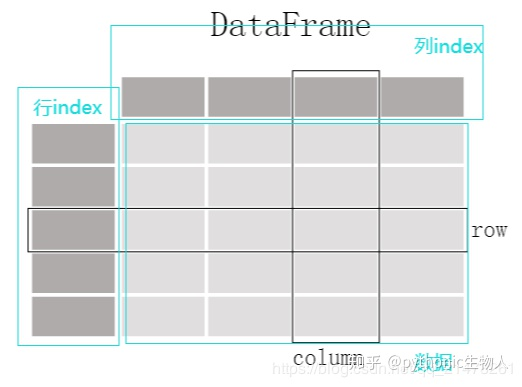DataFame创建
语法：pandas.DataFrame(data=None, index: Optional[Collection] = None, columns: Optional[Collection] = None, dtype: Union[str, numpy.dtype, ExtensionDtype, None] = None, copy: bool = False)dataindex：可选项，行标签columns：可选项，列标签dtype：可选项，元素数据类型
创建方式很多，罗列两种：

#使用字典创建pandas.DataFame
In : d = {'col1': [1, 2], 'col2': [3, 4]}
...: df = pd.DataFrame(d,dtype=np.int8)#dtype指定元素数据类型
In : df
Out:
col1  col2
0     1     3
1     2     4

In : df.dtypes#查看数据类型
Out:
col1    int8
col2    int8
dtype: object

In : df
Out:
col1  col2
0     1     3
1     2     4

#使用二维numpy.ndarry创建pandas.DataFame
In : df2 = pd.DataFrame(np.array([[1, 2, 3], [4, 5, 6], [7, 8, 9]]),
...:                    columns=['a', 'b', 'c'])# columns指定列标签

In : df2
Out:
a  b  c
0  1  2  3
1  4  5  6
2  7  8  9


pandas.DataFrame中取列操作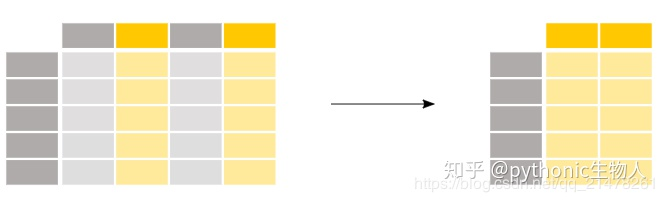#创建实验DataFrame
In : d = {'one': pd.Series([2., 2., 3.,4.], index=['a', 'b', 'c','d']),'two': pd.Series([1., 2., 3., 4.], index=['a', 'b', 'c', 'd']),'three': pd.Series([3., 1., 3., 4.], index=['a', 'b', 'c', 'd'])}
In : df = pd.DataFrame(d)
In : df
Out:
one  two  three
a  2.0  1.0    3.0
b  2.0  2.0    1.0
c  3.0  3.0    3.0
d  4.0  4.0    4.0


pandas.DataFrame中取某一列

In : df['one']
Out:
a    2.0
b    2.0
c    3.0
d    4.0
Name: one, dtype: float64


pandas.DataFrame中取某几列-方法1

In : df[['one','three']]#[[]]，中括号中放入一个1索引值列表
Out:
one  three
a  2.0    3.0
b  2.0    1.0
c  3.0    3.0
d  4.0    4.0


pandas.DataFrame中取某几列-方法2

In : df.iloc[:,[0,2]]#df.iloc[行索引, [列索引号]]
Out:
one three
a 2.0 3.0
b 2.0 1.0
c 3.0 3.0
d 4.0 4.0


pandas.DataFrame中取行操作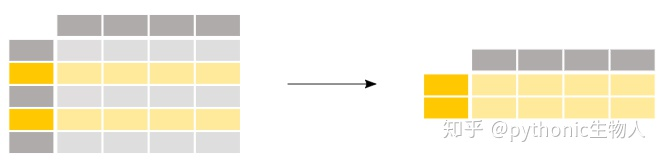pandas.DataFrame中取某一行

In : df
Out:
one two three
a 2.0 1.0 3.0
b 2.0 2.0 1.0
c 3.0 3.0 3.0
d 4.0 4.0 4.0

In : df.loc[['a']]
Out:
one two three
a 2.0 1.0 3.0
In : type(df.loc[['a']])#返回一个DataFrame对象
Out: pandas.core.frame.DataFrame

In : df.loc['a']#使用['a']和[['a']]呈现方式有区别
Out:
one 2.0
two 1.0
three 3.0
In : type(df.loc['a'])#返回一个Series对象
Out: pandas.core.series.Series


pandas.DataFrame中取某几行

In : df.loc[['a','d']]
Out:
one two three
a 2.0 1.0 3.0
d 4.0 4.0 4.0


pandas.DataFrame中按一个条件过滤行
此时传入布尔向量辅助过滤。

In : df
Out:
one two three
a 2.0 1.0 3.0
b 2.0 2.0 1.0
c 3.0 3.0 3.0
d 4.0 4.0 4.0

In : df['two']#取出'two'列
Out:
a 1.0
b 2.0
c 3.0
d 4.0
Name: two, dtype: float64

In : df['two'] > 2#'two'列判断返回布尔型Series对象
Out:
a False
b False
c True
d True
Name: two, dtype: bool

In : df[df['two'] > 2]#取出'two'列中元素值大于2的行
Out:
one two three
c 3.0 3.0 3.0
d 4.0 4.0 4.0


pandas.DataFrame中按多个条件过滤行
此时会用到逻辑运算符：

| 或者& 且~ 取反

注意使用括号将每个条件括起来，如下：

In : df[(df['two'] >= 2) & (df['three'] >= 3)]#取'two'列大于等于2且'three'列大于等于3的行
Out:
one two three
c 3.0 3.0 3.0
d 4.0 4.0 4.0


pandas.DataFrame中结合map 和lambda函数过滤行

In : df
Out:
one two three
a 2.0 1.0 3.0
b 2.0 2.0 1.0
c 3.0 3.0 3.0
d 4.0 4.0 4.0

In : fileter_ = df['one'].map(lambda x: str(x).startswith('2'))#取出'one'列中以2开头的字符
In : df[fileter_]
Out:
one two three
a 2.0 1.0 3.0
b 2.0 2.0 1.0


pandas.DataFrame中结合isin过滤行

In : df
Out:
one two three
a 2.0 1.0 3.0
b 2.0 2.0 1.0
c 3.0 3.0 3.0
d 4.0 4.0 4.0

In : df['one'].isin([1,2])#取出'one'列中值为1或者2的行
Out:
a True
b True
c False
d False
Name: one, dtype: bool

In : df[df['one'].isin([1,2])]
Out:
one two three
a 2.0 1.0 3.0
b 2.0 2.0 1.0


pandas.DataFrame中取某几行中的某几列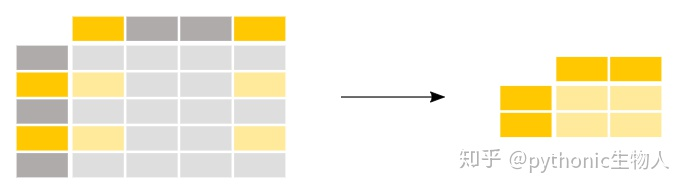In : df
Out:
one two three
a 2.0 1.0 3.0
b 2.0 2.0 1.0
c 3.0 3.0 3.0
d 4.0 4.0 4.0

In : df.iloc[[0,3],[0,1]]#取第0行，3行的第1列和第2列
Out:
one two
a 2.0 1.0
d 4.0 4.0


pandas.DataFrame中替换值：where方法
where对不满足条件的元素执行操作，不会改变原数据。

In : df
Out:
one two three
a 2.0 1.0 3.0
b 2.0 2.0 1.0
c 3.0 3.0 3.0
d 4.0 4.0 4.0

In : df.where(df['one'] < 3, df-1)#对于'one'列元素大于等于3的行（c和d行）执行减1操作
Out:
one two three
a 2.0 1.0 3.0
b 2.0 2.0 1.0
c 2.0 2.0 2.0
d 3.0 3.0 3.0


pandas.DataFrame中查找某个位置的值：lookup方法
（个人感觉没什么卵用）

In : df
Out:
one two three
a 2.0 1.0 3.0
b 2.0 2.0 1.0
c 3.0 3.0 3.0
d 4.0 4.0 4.0

In : df.lookup(['d','a'],['one','three'])#查找坐标为（'d','one'）和('a','three')的值
Out: array([4., 3.])

In : df.lookup(['a','d'],['one','three'])
Out: array([2., 4.])


pandas.DataFrame中末尾新增加列
会直接修改原始数据，慎重使用。

In : df1
Out:
one two three
a 2.0 1.0 3.0
b 2.0 2.0 1.0
c 3.0 3.0 3.0
d 4.0 4.0 4.0

In : df1['five'] = -df1['three']#默认在末尾添加以列
In : df1
Out:
one two three five
a 2.0 1.0 3.0 -3.0
b 2.0 2.0 1.0 -1.0
c 3.0 3.0 3.0 -3.0
d 4.0 4.0 4.0 -4.0


pandas.DataFrame中指定位置新增加列：insert方法

In : df.insert(1, 'new_one', df['one'])#在第一列后新增加列
In : df
Out:
one new_one two three
a 2.0 2.0 1.0 3.0
b 2.0 2.0 2.0 1.0
c 3.0 3.0 3.0 3.0
d 4.0 4.0 4.0 4.0


pandas.DataFrame中删除列：del方法
会直接修改原始数据，慎重使用。

In : del df1['five']
In : df1
Out:
one two three
a 2.0 1.0 3.0
b 2.0 2.0 1.0
c 3.0 3.0 3.0
d 4.0 4.0 4.0


pandas.DataFrame复制：copy方法
可以避免del，insert这种方法直接修改原始数据。

In : df1 = df.copy()
In : df1
Out:
one new_one two three
a 2.0 2.0 1.0 3.0
b 2.0 2.0 2.0 1.0
c 3.0 3.0 3.0 3.0
d 4.0 4.0 4.0 4.0

In : del df1['new_one']
In : df1#df1被修改
Out:
one two three
a 2.0 1.0 3.0
b 2.0 2.0 1.0
c 3.0 3.0 3.0
d 4.0 4.0 4.0

In : df#df未被修改
Out:
one new_one two three
a 2.0 2.0 1.0 3.0
b 2.0 2.0 2.0 1.0
c 3.0 3.0 3.0 3.0
d 4.0 4.0 4.0 4.0


In : df
Out:
one new_one two three
a 2.0 2.0 1.0 3.0
b 2.0 2.0 2.0 1.0
c 3.0 3.0 3.0 3.0
d 4.0 4.0 4.0 4.0

Out:
one new_one two three
a 2.0 2.0 1.0 3.0
b 2.0 2.0 2.0 1.0
c 3.0 3.0 3.0 3.0

In : df.tail(3)#查看末尾三行
Out:
one new_one two three
b 2.0 2.0 2.0 1.0
c 3.0 3.0 3.0 3.0
d 4.0 4.0 4.0 4.0


Series、pandas.DataFrame中某列唯一值的个数：unique

In : pd.Series(['c', 'a', 'd', 'a', 'a', 'b', 'b', 'c' , 'c']).unique()
Out: array(['c', 'a', 'd', 'b'], dtype=object)


pandas.DataFrame统计列中每个元素出现的频次：value_counts方法

In : df1
Out:
one two
a 1.0 1.0
b 2.0 2.0
c 3.0 NaN
d NaN 4.0

In : df1['one'].value_counts()
Out:
3.0 1
2.0 1
1.0 1
Name: one, dtype: int64

In : df1['one'].value_counts(dropna=False)#不跳过缺省值
...:
Out:
NaN 1
3.0 1
2.0 1
1.0 1
Name: one, dtype: int64


pandas.DataFrame按照某几列分组并统计：groupby+count

In : df = pd.DataFrame([('bird', 'Falconiformes', 389.0),
...: ('bird', 'Psittaciformes', 24.0),
...: ('mammal', 'Carnivora', 80.2),
...: ('mammal', 'Primates', np.nan),
...: ('mammal', 'Carnivora', 58)],
...: index=['falcon', 'parrot', 'lion', 'monkey', 'leopard'],
...: columns=('class', 'order', 'max_speed'))

In : df
Out:
class           order  max_speed
falcon     bird   Falconiformes      389.0
parrot     bird  Psittaciformes       24.0
lion     mammal       Carnivora       80.2
monkey   mammal        Primates        NaN
leopard  mammal       Carnivora       58.0

In : df.groupby(['class','order']).count()
Out:
max_speed
class  order
bird   Falconiformes           1
Psittaciformes          1
mammal Carnivora               2
Primates                0


pandas.DataFrame按照某列分组并求和：

In : df2 = pd.DataFrame({'X': ['B', 'B', 'A', 'A'], 'Y': [1, 2, 3, 4]})
In : df2
Out:
X Y
0 B 1
1 B 2
2 A 3
3 A 4

In : df2.groupby(['X']).sum()
Out:
Y
X
A 7
B 3

In : df2.groupby(['X'],sort=False).sum()
Out:
Y
X
B 3
A 7


pandas.DataFrame按照某列分组并取出某个小组：groupby+get_group

In : df2.groupby(['X']).get_group('A')
Out:
X Y
2 A 3
3 A 4

#统计groupby分组的组数
In : len(df2.groupby(['X'],sort=False).get_group('A'))
Out: 2


pandas.DataFrame排序
pandas.DataFrame按照行标签或者列标签排序：sort_index方法
默认为升序，ascending可设置排序方式。

In : frame = pd.DataFrame(np.arange(8).reshape((2, 4)), index=['three', 'one'], columns=['d', 'a', 'b', 'c'])
In : frame
Out:
d a b c
three 0 1 2 3
one 4 5 6 7
In : frame
Out:
d  a  b  c
three  0  1  2  3
one    4  5  6  7

In : frame.sort_index()#行索引排序
Out:
d  a  b  c
one    4  5  6  7
three  0  1  2  3

In : frame.sort_index(axis=1)#列索引排序
Out:
a  b  c  d
three  1  2  3  0
one    5  6  7  4

In : frame.sort_index(axis=1, ascending=False)#列索引排序，ascending设置升序还是降序排序
Out:
d c b a
three 0 3 2 1
one 4 7 6 5


pandas.DataFrame按照某列值排序：sort_values方法by参数

In : frame.sort_values(by='d')#按照d列值排序，默认是升序
Out:
d a b c
three 0 1 2 3
one 4 5 6 7

In : frame.sort_values(by='d',ascending=False)#ascending设置降序排序
Out:
d a b c
one 4 5 6 7
three 0 1 2 3

In : frame.sort_values(by=['c','d'],ascending=False)#按照c,d的值排序，即先按c列值排序，c列值相同时再按d列值排序
Out:
d a b c
one 4 5 6 7
three 0 1 2 3


pandas.DataFrame描述统计

Pandas中描述和汇总统计常用函数
方法  说明
count   非 NA 值的数量
describe    针对 Series 或 DataFrame 的列计算汇总统计，对于数值和字符返回结构不一样
min , max   最小值和最大值
argmin , argmax 最小值和最大值的索引位置（整数）
idxmin , idxmax 最小值和最大值的索引值
quantile    样本分位数（0 到 1）
sum 求和
mean    均值
median  中位数
var 方差
std 标准差
skew    样本值的偏度（三阶矩）
kurt    样本值的峰度（四阶矩）
cumsum  样本值的累计和
cummin , cummax 样本值的累计最大值和累计最小值
cumprod 样本值的累计积
diff    计算一阶差分（对时间序列很有用）
pct_change  计算百分数变化

In : df = DataFrame([[1.4,np.nan],[7.1,-4.5],[np.nan,np.nan],[0.75,-1.3]],index=['a','b','c','d'],columns=['one','two'])
In : df
Out:
one two
a 1.40 NaN
b 7.10 -4.5
c NaN NaN
d 0.75 -1.3

In : df.sum()#默认求每列的和
Out:
one 9.25
two -5.80
dtype: float64

In : df.sum(axis = 1)#传入参数axis，求每行的和
Out:
a 1.40
b 2.60
c 0.00
d -0.55
dtype: float64

In : df.describe()#输出每列值的多个统计指标，对于NaN值直接跳过
Out:
one two
count 3.000000 2.000000
mean 3.083333 -2.900000
std 3.493685 2.262742
min 0.750000 -4.500000
25% 1.075000 -3.700000
50% 1.400000 -2.900000
75% 4.250000 -2.100000
max 7.100000 -1.300000

In : pd.Series(['a', 'a', 'b', 'c']).describe()
Out:
count 4
unique 3#字符去重后的个数
top a
freq 2
dtype: object


pandas.DataFrame获取行列标签/表头名称

In : df
Out:
one two
a 1.40 NaN
b 7.10 -4.5
c NaN NaN
d 0.75 -1.3

In : list(df.columns.values)#列标签
Out: ['one', 'two']

In : list(df.index.values)#行标签
Out: ['a', 'b', 'c', 'd']


参考资料

https://pandas.pydata.org/pandas-docs/stable/index.html
[Python for Data Analysis, 2nd Edition]
(
https://
pandas.pydata.org/panda
s-docs/stable/index.html
)
Python数据科学手册

欢迎微信随缘关注@pythonic生物人

展开全文• 在数据分析中，经常涉及numpy中的ndarray对象与pandas的Series和DataFrame对象之间的转换，让一些开发者产生了困惑。本文将简单介绍这三种数据类型，并以金融市场数据为例，给出相关对象之间转换的具体示例。ndarray...


在数据分析中，经常涉及numpy中的ndarray对象与pandas的Series和DataFrame对象之间的转换，让一些开发者产生了困惑。本文将简单介绍这三种数据类型，并以金融市场数据为例，给出相关对象之间转换的具体示例。
ndarray数组对象
NumPy中的ndarray是一个多维数组对象，该对象由两部分组成：
实际的数据；
描述这些数据的元数据。
大部分的数组操作仅仅修改元数据部分，而不改变底层的实际数据。NumPy数组一般是同质的(但有一种特殊的数组类型例外，它是异质的)，即数组中的所有元素类型必须是一致的。这样有一个好处：如果我们知道数组中的元素均为同一类型，该数组所需的存储空间就很容易确定下来。
我们举一个简单的多维数组的例子。在这里，我们使用Numpy提供的arange函数来来方便的创建一维数组，其函数原型为arange([start,] stop[, step,], dtype=None)。可以指定起始值、结束值、步长及数据类型参数，这里步长参数为整数值。如果要使用非整数值的步长，可以考虑使用linespace函数。通过reshape()函数将一维数组转换为多维数组。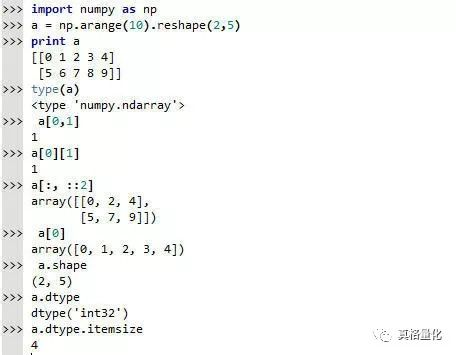多维数组的操作也非常简单，可以参考list类型对数组进行访问、切片操作。比较重要的是可以通过shape属性获取数组的维数。
我们也可以通过多维数组来标识异构的数据类型，以股票价格数据为例，通过dtype类来定义数据类型对象stock, 其包括日期、开盘价、收盘价、最高价、最低价、成交量及股票编码信息：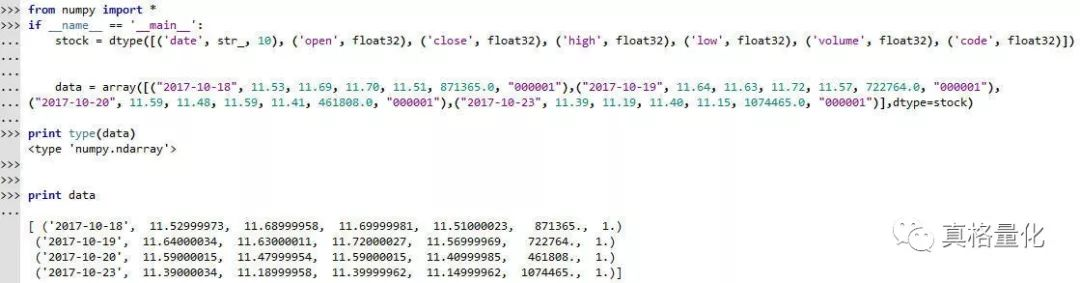不过在实际应用中，我们很少使用ndarray来定义异构的数据类型，而是使用pandas中的Series和DataFrame来操作。
ndarray的性能优势
一、内存块风格:
这是因为ndarray中的所有元素的类型都是相同的，而Python列表中的元素类型是任意的，所以ndarray在存储元素时内存可以连续，而python原生lis就t只能通过寻址方式找到下一个元素，这虽然也导致了在通用性能方面Numpy的ndarray不及Python原生list，但在科学计算中，Numpy的ndarray就可以省掉很多循环语句，代码使用方面比Python原生list简单的多。
二、ndarray支持并行化运算(向量化运算)。
三、Numpy底层使用C语言编写，内部解除了GIL(全局解释器锁)，其对数组的操作速度不受Python解释器的限制，效率远高于纯Python代码。
Series对象
从一般意义上来讲， Series 可以简单地被认为是一维的数组。Series 和一维数组最主要的区别在于 Series 类型具有索引( index )。Series支持从列表和字典创建，这里仅举以列表创建的例子：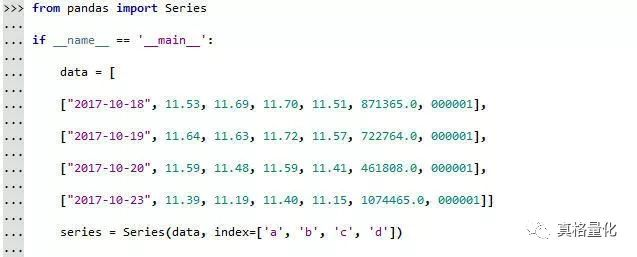输出结果为：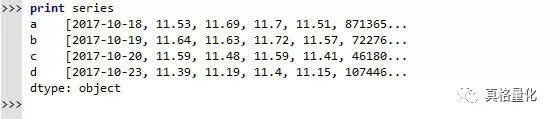我们还可以将Series转换为ndarray类型：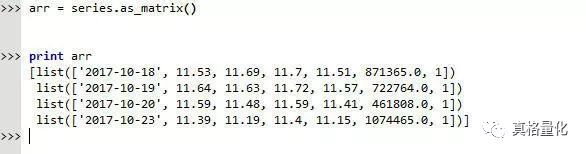或者使用.values。
DataFrame对象
DataFrame 是将数个 Series 按列合并而成的二维数据结构，每一列单独取出来是一个 Series ，这和SQL数据库中取出的数据是很类似的。所以，按列对一个 DataFrame 进行处理更为方便，开发者在编程时注意培养按列构建数据的思维。DataFrame 的优势在于可以方便地处理不同类型的列，因此，就不要考虑如何对一个全是浮点数的 DataFrame 求逆之类的问题了，处理这种问题还是把数据存成NumPy的 matrix 类型比较便利一些。
我们仍用一个股票价格数据的例子，创建DataFrame对象，这里把日期提取出来作为index，同时指定了列名。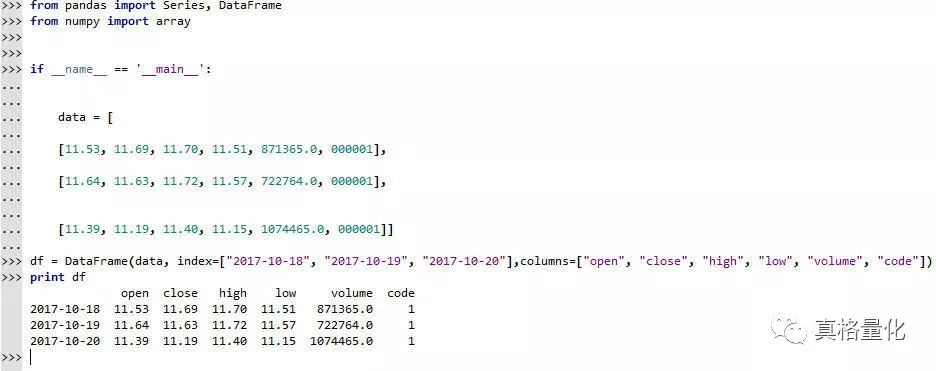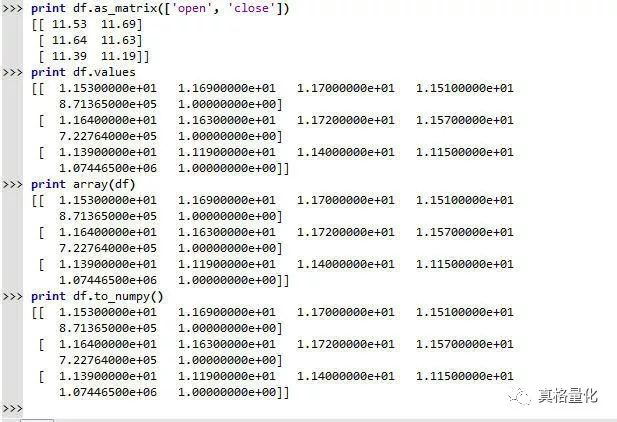这里，我们展示了4种方法将DataFrame转化为ndarray类型的方法。as_matrix()方法可以指定获取的列；values属性将使用所有的列转换为ndarray对象，等同于无参数的as_matrix()；array()接受将DataFrame对象作为参数创建ndarray对象。to_numpy()也是将DataFrame转为ndarray对象。
— — — — — — E N D — — — — — —
真格量化可访问：
https://quant.pobo.net.cn真格量化微信公众号，长按关注：遇到了技术问题？欢迎加入真格量化Python技术交流QQ群  726895887往期文章：
Numpy处理tick级别数据技巧
真正赚钱的期权策略曲线是这样的
多品种历史波动率计算
如何实现全市场自动盯盘
AI是怎样看懂研报的
真格量化策略debug秘籍
真格量化对接实盘交易
常见高频交易策略简介
如何用撤单函数改进套利成交
Deque提高处理队列效率
策略编程选Python还是C++
如何用Python继承机制节约代码量
十大机器学习算法
如何调用策略附件数据
如何使用智能单
如何扫描全市场跨月价差
如何筛选策略最适合的品种
活用订单类型规避频繁撤单风险
真格量化回测撮合机制简介
如何调用外部数据
如何处理回测与实盘差别
如何利用趋势必然终结获利
常见量化策略介绍
期权交易“七宗罪”
波动率交易介绍
推高波动率的因素
波动率的预测之道
趋势交易面临挑战
如何构建知识图谱
机器学习就是现代统计学
AI技术在金融行业的应用
如何避免模型过拟合
低延迟交易介绍
架构设计中的编程范式
交易所视角下的套利指令撮合
距离概念与特征识别
气象风险与天气衍生品
设计量化策略的七个“大坑”
云计算在金融行业的应用
机器学习模型评估方法
真格量化制作期权HV-IV价差
另类数据介绍
TensorFlow中的Tensor是什么？
机器学习的经验之谈
用yfinance调用雅虎财经数据
容器技术如何改进交易系统
Python调用C++
如何选择数据库代理
统计套利揭秘
一个Call搅动市场？让我们温习一下波动率策略
如何用真格量化设计持仓排名跟踪策略
还不理解真格量化API设计？我们不妨参考一下CTP平台
理解同步、异步、阻塞与非阻塞
隐波相关系数和偏度——高维风险的守望者
Delta中性还不够？——看看如何设计Gamma中性期权策略
Python的多线程和多进程——从一个爬虫任务谈起
线程与进程的区别皮尔逊相关系数与历史K线匹配
Python2和Python3的兼容写法Python代码优化技巧
理解Python的上下文管理器
如何写出更好的Python代码？这是Python软件基金会的建议
评估程序化模型时我们容易忽视的指标
看看如何定位Python程序性能瓶颈
什么是Python的GIL
投资研究中的大数据分析趋势及应用
理解CTP中的回调函数
如何围绕隐含波动率设计期权交易策略
看看如何用Python进行英文文本的情感分析
算法交易的分类
Python编码的最佳实践总结
什么是波动率锥？如何用波动率锥设计期权策略？
期权的波动率策略与时间价值收集策略对比
期权用于套期保值和无风险套利
隐含波动率对期权策略的影响
卖出期权交易的风险管理原则和技巧
期权交易中的“大头针”风险
期权做市商策略简介
精细化您的交易——交易成本评估与交易执行策略
海外市场交易执行策略的实践
设计期权套期保值方案时应注意的问题
美式期权、欧式期权比较分析——定价与风险管理
构建您的AI时代武器库——常用的机器学习相关Python库
期权波动率“微笑曲线”之谜
运算任务愈发繁重，如何加速Python程序运行？
证券市场微观结构理论模型是什么
是瞬间成交还是漫长等待？——如何衡量市场流动性
波动率指数及其衍生品介绍
Python的异常处理技巧
Python中的阻塞、异步与协程
"香草"之外的更多选择——几种常见的路径依赖奇异期权
什么是CTP？——了解上期所CTP快速交易系统
了解季节性——以谷物和油籽为例
是前因还是后果?——在真格量化中进行格兰杰因果检验
Python导入模块的技巧
Python程序员常犯的十个错误
搜索数据泄露天机？——舆情指数与期货行情关联性分析思路
机器学习常见算法分类汇总
如何使用Data Pipeline 自动化数据处理工作？
CTP API的委托介绍和在真格量化中的订单流控制
高频交易对市场的影响
期货行情及其组织形式——以上期所为例
理解并行与并发
郑商所和大商所套利指令及在真格量化的实现
机器学习用于金融市场预测面临的挑战
高频交易中风险控制的常用措施
查询结果偏离预期？来了解CTP的报单函数及委托状态查询
Python中的ftplib模块
理解真格量化的Python编程范式
需要处理大量市场数据？来了解一下MySQL、HBase、ES的特点和应用场景


展开全文• 下面介绍一种更改DataFrame中某一列数据的方法: 现将这一列数据通过列索引提取出来(此时提出来的部分为Series对象), 然后用map方法进行修改. (DataFrame中的applymap方法用得还不熟, 等研究过了再试试有没有另一种...
        下面介绍一种更改DataFrame中某一列数据的方法: 现将这一列数据通过列索引提取出来(此时提出来的部分为Series对象), 然后用map方法进行修改. (DataFrame中的applymap方法用得还不熟, 等研究过了再试试有没有另一种方法)
代码: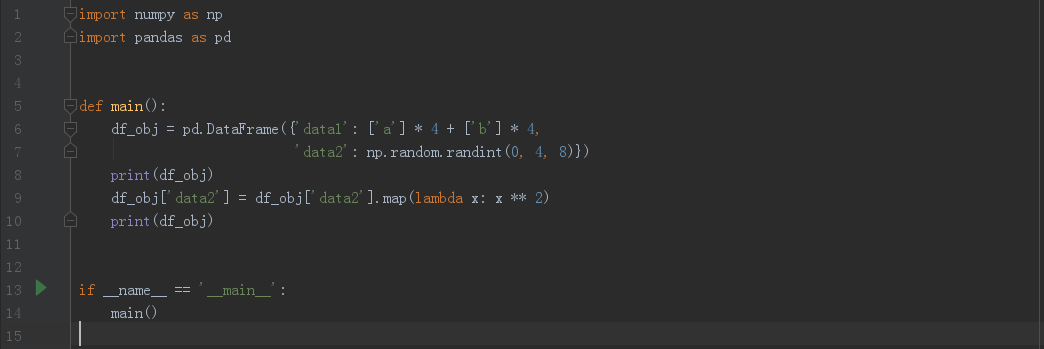输出结果: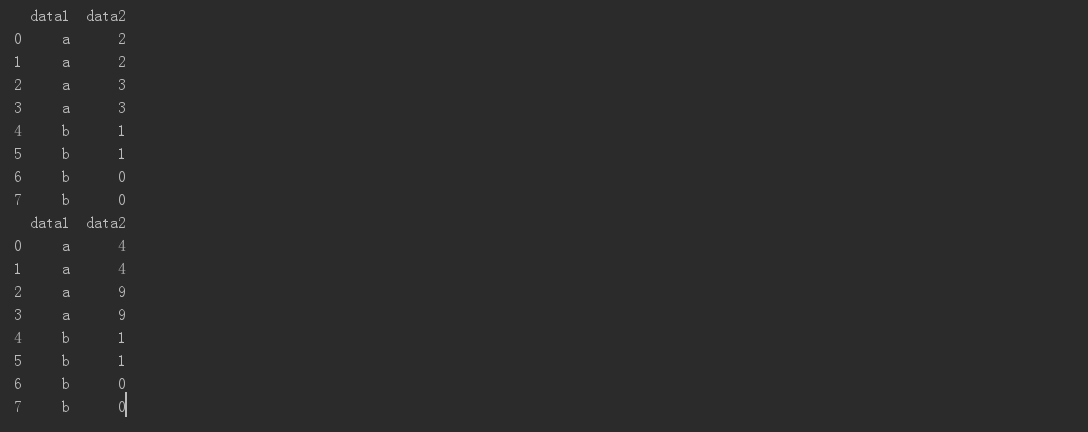展开全文pandas
• 1 pandas简介1.Pandas是什么...2.DataFrameDataFrame是Pandas中的个表格型的数据结构，包含有组有序的，每可以是不同的值类型(数值、字符串、布尔型等)，DataFrame即有行索引也有索引，可以被看做是由Ser...

1 pandas简介
1.Pandas是什么？
Pandas是一个强大的分析结构化数据的工具集；
它的使用基础是Numpy（提供高性能的矩阵运算）；
用于数据挖掘和数据分析，同时也提供数据清洗功能。
2.DataFrame
DataFrame是Pandas中的一个表格型的数据结构，包含有一组有序的列，每列可以是不同的值类型(数值、字符串、布尔型等)，DataFrame即有行索引也有列索引，可以被看做是由Series组成的字典。
pandas 相当于 python 中 excel：它使用表（也就是 dataframe)，能在数据上做各种变换，但还有其他很多功能。

class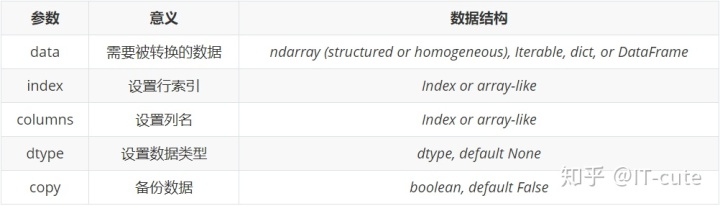3.Series
它是一种类似于一维数组的对象，是由一组数据(各种NumPy数据类型)以及一组与之相关的数据标签(即索引)组成。仅由一组数据也可产生简单的Series对象。
4.pandas 网址
官网：https://pandas.pydata.org/
中文网：https://www.pypandas.cn/
2 导入
符号含义df二维的表格型数据结构DataFrames一维数组Series （一个一维的标签矩阵）
还需要执行以下导入才能开始：

import 

3 使用

# 使用Pandas


4 读取、写入

import 


展开全文• dataframe对象获取切片的常用方法有两种，种是通过索引名和列名，即使用.loc ,另外种是使用行号和号，即使用.iloc 。 例如下述dataframe； import pandas as pd import numpy as np data=np.around(np.random....
• 对于数据科学家，无论是数据分析还是数据挖掘来说，Pandas是个非常重要的Python包。它不仅提供了很多方法，使得数据处理非常简单，同时在数据处理速度上也做了很多优化，使得和Python内置方法相...
• 问题的由来：我想用seanborn的kdeplot画图，kdeplot第个参数需要是维数组。 我开始传入的是Dataframe类变量，这个Dataframe类变量是使用过Dataframe[['列名']]取得，然后就报iloc giving 'IndexError: ...pandas Dataframe Python
• 1 Serise对象Series维数组对象 ，它包含组索引和组数据，可以把它理解为组带索引的数组。&gt;&gt;&gt; from pandas import Series,DataFrame &gt;&gt;&gt; series =...python
• Pandas是Python下最强大的数据分析和探索库，是基于Numpy库构建的，支持类似SQL的结构化数据的增、删、查、改，具有丰富的数据处理函数。Pandas有两大数据结构：...Series对象本质上类似于一个一维数组，由一列元...
• 一个 DataFrame 取一列，不同的表示方法，名字是不是列表，会影响得到的结果的类型series 或者 DataFrame.  python果真是好入手，不好学精呀，之前一直知道取一列后类型会改变问serious,每次都要小心的进行处理，...DataFrame
• python pandas 如何替换某列的一个值摘要：本文主要是讲解怎么样替换某一列的一个值。应用场景：假如我们有以下的数据集：我们想把里面不是pre的字符串全部换成Nonpre，我们要怎么做呢？做法很简单。df['col2']=df['...
• python中对已经生成的Series，怎样组合成DataFrame如 a = Series([1,2,3]) b = Series([2,3,4]) 怎样将a b组合成一个DataFzip函数接受任意多个（包括0个和1个）序列作为参数，返回个tuple列表。具体意思不好用文字...
• #为Series对象,返回索引为原DataFrame的索引，等价于d1.age#0 241 652 343 804 125 28Name: age, dtype: int64#取行d1.ix#age 80city ShangHaigender 0Name: 3, dtype: objectd1.loc #取行做使用.loc或者....
• 1.对excel进行操作，对查找的内容进行精准匹配...from pandas import Series,DataFrame test=pd.read_excel('测试.xlsx',sheet_name='test') #读入需要处理的表格及sheet页 #test list=['小烟','想睡觉','天真好','...python excel 正则表达式 大数据
• 目录Python之pandas中Series对象下的str方法汇总1. cat（和指定字符进行拼接）2. split（按照指定字符串分隔）3. rsplit（和split用法一致，只不过默认是从右往左分隔）4. partition（也是按照指定字符串分隔，和...python
• filename, col_name): """读取某一列""" with open(filename, 'r', encoding='utf-8') as file: reader = csv.DictReader(file) column = [row[col_name] for row in reader if row[col_name]] return column def ...
• 在Pandas中，DataFrame的一列就是一个Series, 可以通过map来对一列进行操作： 例如，将df['age']列的所有元素进行平方操作，可以使用： df['age'] =df['age'].map(lambda x: x**2)
• 文章目录【01x00】了解 Pandas【01x01】Axes3D 对象创建方法：Axes3D(fig) 这里是段防爬虫文本，请读者忽略。 本文原创首发于 CSDN，作者 TRHX。 博客首页：https://itrhx.blog.csdn.net/ 本文链接：...DataFrame python 数据分析 pandas
• 今天小编就为大家分享篇从DataFrame中提取出Series或DataFrame对象的方法，具有很好的参考价值，希望对大家有所帮助。一起跟随小编过来看看吧
• .操作excle文件 1.1 读取excle 1.1.1 read_excle data = pandas.read_excel(io, sheet_name=0, header=0, names=None, index_col=None, usecols=None, squeeze=False,dtype=None, engine=None, ...
• pandas中的DataFrame是我们在数据分析时最常用到的类数据结构，而在数据分析时，对数据进行排序也是经常用的种操作，因此，本文将针对DataFrame的排序问题进行个详细的说明。  对DataFrame对象排序可以分为...
• 其实只要聚合的时候指定分组的不作为index就行。 action.groupby([&amp;#39;sku_id&amp;#39;, &amp;#39;type&amp;#39;], as_index=False).count()添加as_index=False
• 图表中所有点的数据都来自数据列对象，数据的基本构造是： series : [{ name : '', data : [] }] 提示：数据配置是个数组，也就是数据配置可以包含多个数据。 数据中的 name 代表数据的名字，...
• Pandas数据处理 ...当在两个Series或DataFrame对象上进行二元计算时，Pandas会在计算过程中对齐两个对象的索引。 Series索引对齐 A=pd.Series([2,4,6],index=[0,1,2]) B=pd.Series([1,3,5],index=...
• 在使用pandas的时候，经常要对DataFrame的某一列进行操作，一般都会使用df["xx"].str下的方法，但是都有哪些方法呢？我们下面来罗列并演示一下。既然是df["xx"].str，那么xx这一列必须是字符串类型，当然在pandas...
• 数据配置是 Highcharts 最复杂也是最灵活的配置，如果说 Highcharts 是灵活多变，细节可定制的话，那么...图表中所有点的数据都来自数据列对象，数据的基本构造是： series : [{ name : '', data : [] }] 提...